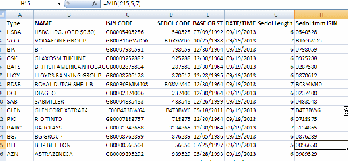## Sedol codes & looking for dataRecently I noticed something odd about Sedol codes that I downloaded using Datastream: not all codes consisted of the same number of characters! Sedol codes should consist of 7 characters. Older codes may have zeros added in front to complete the code. An example is the Sedol code for BHP Billiton: 0056650. When I download the Sedol code in Datastream using a static request the resulting code that I get is: 56650.
If you try to upload this abbreviated code again in databases loke Datastream, Compustat Global or SDC Platinum, this will cause errors or you will not find data on companies using the abbreviated codes. There are two ways to handle the situation.

The easiest way out is if you also downloaded the ISIN codes for the equities/company listings. In the example BHP Billiton the ISIN code of the listing at the London Stock Exchange is: GB0000566504. The Sedol is actually the main constituent of the ISIN code! Using the Excel MID() function you could get the Sedol codes from the ISIN codes. If the ISIN code is in column C in Excel you might say: =MID(C15,5,7). What the Mid() function does is: you tell it to take 7 characters from the contents of cell C15 starting with the fifth character. Dutch language version: =DEEL(C15;5;7)

Example screenshot:The alternative way should only be used when necessary, because it is a bit more dificult to implement. When you have no way out, and you only have abbreviated Sedol codes to continue, you might try the following: use the Excel nesting principle and the formula IF() in combination with the function Length LEN().

The function IF works as follows: =IF(“if condition stated is true”, then “this value, else if(“if this condition stated here is true”, then “this value, else “this value”)).
To make it easier to work with the IF condition, you can divide up the conditions again in separate rows and afterwards make it a single string again:
=IF(B4<=44,”F”,
IF(B4<=54,”D”,
IF(B4<=74,”C”,
IF(B4<=89,”B”,
“A”))))

Full string example:
=IF(B4<=44,”F”,IF(B4<=54,”D”,IF(B4<=74,”C”,IF(B4<=89,”B”,”A”))))

If we have a large list of Sedol codes, you do not want to manually go through it to find out how long each code is and then tell Excel to add zeros to create 7-character Sedol codes again. The first thing you do is work out the conditions based on an abbreviated code (in this example the Individual conditions necessary to get the original 7 digit Sedol. Basically, each line says: If the length of the code in Cell C2 is 6, You need to add a zero, or else show the original code (assuming the length of the code would then be 7). Unfortunately you need to create more conditions for when the code is shorter:
=(IF(LEN(C2)=6,”0″&(C2),(C2)))
=(IF(LEN(C2)=5,”00″&(C2),(C2)))
=(IF(LEN(C2)=4,”000″&(C2),(C2)))
=(IF(LEN(C2)=3,”0000″&(C2),(C2)))
=(IF(LEN(C2)=2,”00000″&(C2),(C2)))
=(IF(LEN(C2)=1,”000000″&(C2),(C2)))

Now you can combine the arguments using the IF function to get a single formula. In this case for the 7 digit Sedol solution:
=(IF(LEN(C2)=6,”0″&(C2),
(IF(LEN(C2)=5,”00″&(C2),
(IF(LEN(C2)=4,”000″&(C2),
(IF(LEN(C2)=3,”0000″&(C2),
(IF(LEN(C2)=2,”00000″&(C2),
(IF(LEN(C2)=1,”000000″&(C2),
(C2)))))))))))))

The full string would then be (working Example):
=(IF(LEN(C2)=6,”0″&(C2),(IF(LEN(C2)=5,”00″&(C2),(IF(LEN(C2)=4,”000″&(C2),(IF(LEN(C2)=3,”0000″&(C2),(IF(LEN(C2)=2,”00000″&(C2),(IF(LEN(C2)=1,”000000″&(C2),(C2)))))))))))))

Example screenshot:Dutch language version of this working example:
=(ALS(LENGTE(C2)=6;”0″&(C2);(ALS(LENGTE(C2)=5;”00″&(C2);(ALS(LENGTE(C2)=4;”000″&(C2);(ALS(LENGTE(C2)=3;”0000″&(C2);(ALS(LENGTE(C2)=2;”00000″&(C2);(ALS(LENGTE(C2)=1;”000000″&(C2);(C2)))))))))))))

An Excel sheet with examples of both solutions can be downloaded here.Email

## WorldScope coverage updateWorldScope company records now cover annual reports data for (currently) 75099 companies. This includes 46563 active and 28536 inactive companies. This update: 256 companies were added. Despite these additions the number of active companies in the database has gone down from 46693 in April. This is the third update in a row that the total number of active companies went down. WorldScope company records are available through Datastream and LexisNexis.

Today I have updated the WorldScope country coverage file and it now includes the latest update as it was posted in the third Thomson Reuters Infostream quarterly publication of 2013.

Major updated Countries (new records):
Canada (18)
Japan (14)
United Kingdom (19)
United States (126)Email